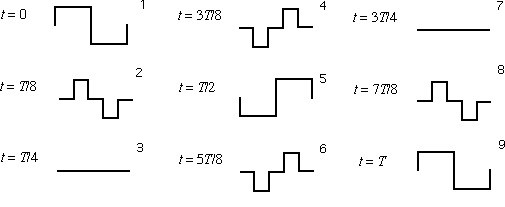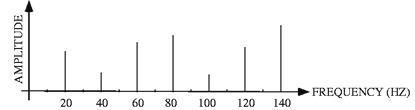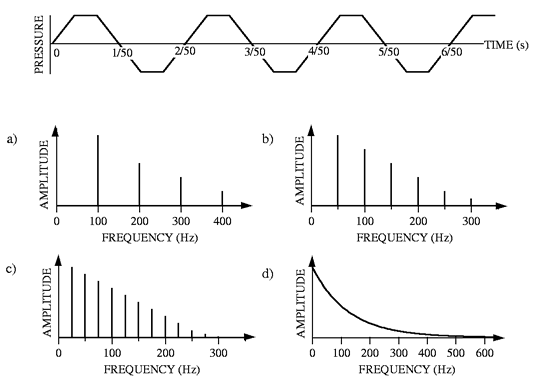Back to "Answer-This" Listing "Answer This" Questions Chapter 7 7-C. Square wave shapes. Explain why the wave shape is flat (zero displacement) at t = T/4 and 3T/4 in the figure. Why is it the negative of its original shape at t = T/2?Answer: A complex wave vanishes at a particular point in space at a certain time either because a) all of its sinusoidal components vanish there at that time, or b) because some of the various components that are positive at that point and time cancel those that are negative at that point and time. In the case of the wave vanishing everywhere at say T/4, a) is the situation. The wave is antisymmetric and is made up only of even harmonics—indeed in this case, as shown in the text, it is made up of only the second harmonic, the sixth harmonic, the tenth harmonic, etc. The repeat time T in this illustration is the period of the second harmonic. By time T/4, the second harmonic component has gone through 1/4 of its period and vanishes everywhere; the sixth harmonic has gone through 3/4 of its period and vanishes everywhere; the tenth harmonic has gone through 5/4 = 1.25 cycles and vanishes everywhere, etc. Such a situation recurs another half cycle T/2 later, i.e., at 3T/4, etc. Similarly after T/2 every harmonic is the negative of what it was at t = 0 and the entire wave is the negative of what it was. An entirely different approach is possible: One can think of the standing square wave as made up of two traveling square waves, one moving to the right and the other moving to the left. (Indeed the animation in this web site was made by using this idea.) The two waves have half the amplitude of the total standing wave; when they are in phase they add and the standing wave is its original shape or its negative; when they slide 180o out of phase, they cancel everywhere. This situation is completely analogous to the situation shown in the standing sinusoidal wave animation.   ____________________________________ 7-D. Repeat time of a complex wave. What is the repeat time of a complex wave made up by adding together three sinusoidal waves having frequencies 18 Hz, 24 Hz, and 60 Hz? Answer: The largest common factor of the three numbers 18, 24, and 60 is 6 (18 = 3x6, 24 = 4x6, 60 = 10x6). So the repeat frequency is 6 Hz and the repeat time is 1/6 s.   ____________________________________ 7-E. Repeat time of a filtered complex wave. A wave having the frequency spectrum shown is passed through a filter that removes all waves below 51 Hz. What is the repeat frequency of the resulting complex wave?Answer: After the filter acts, the frequencies remaining are the 60, 100, and 140 Hz components. The greatest common divisor of those frequencies is still 20 Hz, which is the repeat frequency.   ____________________________________ 7-F. Frequency spectrum The figure shows a pressure-time graph of a repetitive traveling sound wave. Which of the accompanying plots is the most likely frequency spectrum for this wave?Answer: c) The wave is repeating and thus requires a discrete spectrum. Answers d) is continuous and so is eliminated. The repeat time is 2/50 s, so the repeat frequency is 50/2 Hz = 25 Hz. Only c) has a spectrum that has a repeat frequency of 25 Hz, with its fundamental of 25 Hz. [Note symmetry does not play a role here because the wave is shown as a function of time, not position. You are seeing the motion of just a single point in space.] Back to "Answer-This" Listing     © William J. Mullin